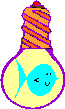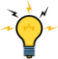# A General Polygon Management RoutineHomeBack To Tips Page

I found myself doing a lot of drawings recently of simple polygons, such as outline shapes. The problem was that these had to be displayed in a variety of rotations. One such shape was a nice compass arrow, which I use in my accompanying essay on doing self-registering custom controls. The complete control is reproduced here. What this essay concentrates on is how I made a nice rotating arrow.The idea here is to create a compass that points to where an object is heading, using geographic coordinates. Much of the detail of the drawing is discussed in the accompanying essay.

To create these simple monochrome shapes, I spent a couple hours writing a trivial editor which allows me to enter a set of x,y coordinates. No mousing, nothing terribly sophisticated, but all I needed was a list of x,y coordinates, not a metafile or anything else elaborate. The most complex drawing I have has about 20 endpoints. I used my little vector editor program, which is described in an accompanying essay.

Here is the set of points that defines the compass needle. The nominal coordinate system is a 100-unit coordinate system ranging ±50.

```-14, 30
-5, 38
0, 46
5, 38
14, 30
4, 34
4, -36
14,-46
0, -40
-14, -46
-4,-36
-4, 34```

## Polygon.h

```class CPolygon
{
public:
CPolygon() { heading = 0.0; scaling = 1.0; }
CRect GetInputBB();
CRgn * GetRgn();
CRect Transform(double angle, double scale);
void Draw(CDC & dc, CDoublePoint pt);
bool IsDefined() { return points.GetSize() > 0; }
protected:
CArray<CDoublePoint, CDoublePoint> points;
double scaling;
CArray<CPoint, CPoint> transformed;
};
```

## CPolygon::CPolygon

CPolygon::CPolygon()

Constructor. Initializes the polygon. The polygon contains no points.

 const CString & filename The name of a file to read.

Reads a set of points from the specified file. If there is an error, returns FALSE. If there is an error, the contents of the points array is not defined. Minimal syntax checking is done on the input, since it is assumed that the input comes from a program.

 UINT resid The resource ID of a POINTS resource LPCTSTR resourcename The name of a POINTS resource

Loads a set of points from a resource segment. The resource segment type must be POINTS. The two forms determine if the name is a numeric resource ID or a string name.

To include a set of points in a resource segment, add the following style of declaration to the \res\projectname.rc2 file:

`resource_name POINTS DISCARDABLE "\\res\\filename"`

For example, to add the arrow resource, I did

`IDP_ARROW POINTS DISCARDABLE "\\res\\arrow.pln"`

Because I did not define a symbol such as

`#define IDP_ARROW 1101`

I use the LPCTSTR version of the method.

Copy the file (in my case, arrow.pln) to the \\res subdirectory of your project.

## CPolygon::GetInputBB

CRect CPolygon::GetInputBB( )

This returns a CRect which is the bounding box of the polygon as it was read in, before any rotational transformation is done to it. The bounding box is the smallest rectangle that completely encloses all the points of an object.

## CPolygon::GetRgn

CRgn * CPolygon::GetRgn( )

Returns the region of the transformed (rotated and scaled) polygon. This can be used to invalidate the area occupied by the object.

## CPolygon::Transform

CRect Transform(double angle, double scale, BOOL force = FALSE)

 double angle The angle of rotation, expressed in geographic coordinates double scale The scaling factor. This is interpreted as relative to the points read in for the definition. BOOL force Forces a recomputation, even if the internal optimizations determine that the existing set of transformed points would be valid.

This transforms the polygon points according to the specified angle (expressed in geographic coordinates, with 0.0 North, 90.0 East, 180.0 South and 270.0 West) and scaling. The result is an internal structure which contains the transformed points, suitable for the Draw method. The CRect returned is the bounding rectangle of the transformed points, or the rectangle (0,0,0,0). As an optimization, if the angle and scale are the same as the previous Transform call, nothing is computed and (0,0,0,0) is returned. If you need to force the recomputation to obtain the bounding box return, use the third parameter, force, and set it TRUE which bypasses the optimization.

Note that the CRect is in mapped coordinates, with y values increasing upwards and x values increasing rightwards; the assumption is that the points are centered around a 0,0 axis which is at the logical center of the object. Therefore, IsRectEmpty will always return TRUE. If you need to check for an empty rectangle, you may need to first make a copy and call the NormalizeRect method on that copy (if you don't need to preserve the CRect, you can call NormalizeRect on the result).

This method must be called before the Draw method is executed. Draw will always draw the polygon based on the current transformation established by Transform. If Transform has not been called since the data was loaded, the transformed points array is empty.

The transformation is a classic rotation around the center point 0,0, according to the transformation matrix shown below. Given two points x,y, a scaling in x sx, and a scaling in y sy, the new points x', y' are computed according to the following matrix multiplication

```             _                   _
|  cos Ø    sin Ø  0  |
[x y 0]|  -sin Ø   cos Ø  0  |=[sx*((cos Ø)*x-(sin Ø)*y)
|_    0     0      0 _|  sy*((sin Ø)*x+(cos Ø)*y) 0]```

(The third column is added to make the matrix multiplication work out, and then discarded).

In my case, I only allow isotropic (sx == sy) scaling.

## CPolygon::IsDefined

BOOL CPolygon::IsDefined( )

Returns TRUE if the polygon is defined by a set of points. Returns FALSE if the array of points is empty. Note that if there is an error in reading, the array of points is usually empty.

## CPolygon::Draw

void CPolygon::Draw(CDC & dc, CDoublePoint pt)

 CDC & dc The DC used to draw the object. CDoublePoint pt The point in the DC, expressed in logical coordinates, at which the image is drawn.

Draws the transformed polygon in the specified DC, at the location specified by the point. The DC is assumed to be in a mapped mode with y increasing upwards (rather than downwards as in MM_TEXT mode). The DC is unmodified upon return. The Transform method must be called before calling Draw to create the set of transformed points. Failing to call Transform will mean that the last transformation will be used; if no transformation has been performed, the transformation set is empty and no drawing will appear.This file can be downloaded as part of the compass example.The views expressed in these essays are those of the author, and in no way represent, nor are they endorsed by, Microsoft.